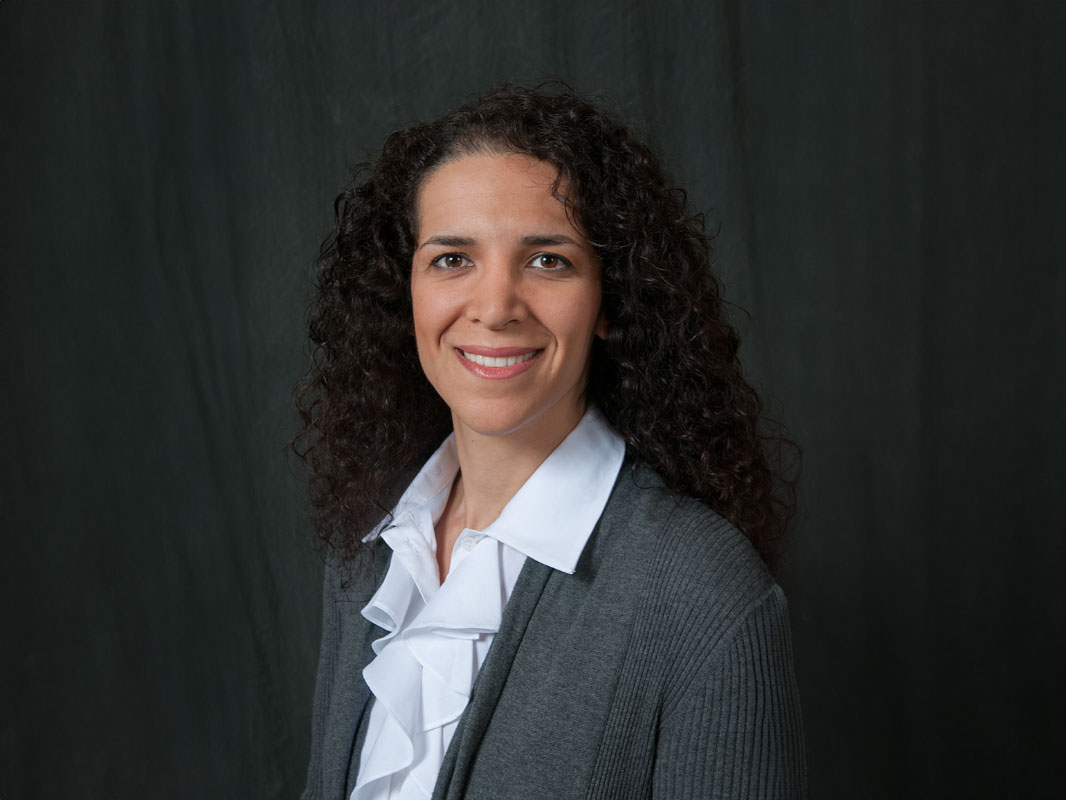# Afsoon Amirzadeh Goghari, PhD

## Full Time Lecturer

### Mechanical Engineering

Curriculum Vitae

508-910-6549

\\hdmu\_`c;ph\nn_)`_p

Textiles 207

## Education

 2010 University of Toronto PhD in Mechanical Engineering
 2006 University of Toronto MASc in Mechanical Engineering
 1999 University of Tehran BSc in Mechanical Engineering

## Teaching

### Courses

Introduction to engineering and computing with emphasis on development of problem solving skills through projects. The course is designed to increase the success of first year students. It includes an overview of majors in the college, and the importance of engaged learning. Team work, written and oral communication skills are covered. Ethical issues in engineering and computing are discussed.

Introduction to engineering and computing with emphasis on development of problem solving skills through projects. The course is designed to increase the success of first year students. It includes an overview of majors in the college, and the importance of engaged learning. Team work, written and oral communication skills are covered. Ethical issues in engineering and computing are discussed.

Introduction to engineering and computing with emphasis on development of problem solving skills through projects. The course is designed to increase the success of first year students. It includes an overview of majors in the college, and the importance of engaged learning. Team work, written and oral communication skills are covered. Ethical issues in engineering and computing are discussed.

Introduction to engineering and computing with emphasis on development of problem solving skills through projects. The course is designed to increase the success of first year students. It includes an overview of majors in the college, and the importance of engaged learning. Team work, written and oral communication skills are covered. Ethical issues in engineering and computing are discussed.

Introduction to engineering and computing with emphasis on development of problem solving skills through projects. The course is designed to increase the success of first year students. It includes an overview of majors in the college, and the importance of engaged learning. Team work, written and oral communication skills are covered. Ethical issues in engineering and computing are discussed.

Introduction to engineering and computing with emphasis on development of problem solving skills through projects. The course is designed to increase the success of first year students. It includes an overview of majors in the college, and the importance of engaged learning. Team work, written and oral communication skills are covered. Ethical issues in engineering and computing are discussed.

Mathematical methods useful to all engineering students. They include: elements of linear algebra, matrices, eigenvalue problems, systems of ordinary differential equations, Fourier series, partial differential equations, probability theory, mathematical statistics, and a brief introduction to complex numbers.MATLAB programming required

Mathematical methods useful to all engineering students. They include: elements of linear algebra, matrices, eigenvalue problems, systems of ordinary differential equations, Fourier series, partial differential equations, probability theory, mathematical statistics, and a brief introduction to complex numbers.MATLAB programming required

Mathematical methods useful to all engineering students. They include: elements of linear algebra, matrices, eigenvalue problems, systems of ordinary differential equations, Fourier series, partial differential equations, probability theory, mathematical statistics, and a brief introduction to complex numbers.MATLAB programming required

Mathematical methods useful to all engineering students. They include: elements of linear algebra, matrices, eigenvalue problems, systems of ordinary differential equations, Fourier series, partial differential equations, probability theory, mathematical statistics, and a brief introduction to complex numbers.MATLAB programming required

## Research

### Research Interests

• Solid Mechanics: Statics and Dynamics
• Mechanical System Design and Control
• Design of Machine Elements
• Renewable Energy
• Fluid Mechanics and Heat Transfer

## Select publications

Amirzadeh, M. Raessi, and S. Chandra (2013).
Producing molten metal droplets smaller than the nozzle diameter using a pneumatic drop-on-demand generator
Exp. Therm. Fluid Sci, 47, 26-33.

Amirzadeh and S. Chandra (2010).
Small droplet formation in a pneumatic drop-on-demand generator: Experiments and analysis
Exp. Therm. Fluid Sci., 34, 1488-1497.

Amirzadeh and S. Chandra (2009).
Analytical and experimental study of small droplet formation in a pneumatic drop-on-demand generator
International Conference on Liquid Atomization and Spray Systems ICLASS, Vail, CO

Afsoon Amirzadeh joined the Mechanical Engineering Department in 2011. She obtained her PhD in Mechanical Engineering from the University of Toronto in 2010. During her graduate studies at the Centre for Advanced Coating Technologies (CACT), Dr. Amirzadeh designed a pneumatic drop-on-demand droplet generator for producing micro-droplets (< 100 microns) of aqueous solutions and molten metals, with applications in bioengineering, rapid prototyping, and micro-soldering. In addition to academic research and teaching, Dr. Amirzadeh gained industrial experience as an experimentalist, and established a climate simulation lab to test auto vehicle parts using international standards.

Latest from Afsoon

Request edits to your profile